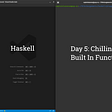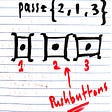# Some Notes on Haskell Pedagogy

`data List a = EmptyList | ListElement a (List a)`
`> testList = ListElement 1 (ListElement 2 (ListElement 3 EmptyList))> testList<interactive>:3:1: error:    • No instance for (Show (List a0)) arising from a use of ‘print’    • In the first argument of ‘print’, namely ‘it’      In a stmt of an interactive GHCi command: print it`
`data List a = EmptyList | ListElement a (List a)  deriving Show`
`> testListListElement 1 (ListElement 2 (ListElement 3 EmptyList))`
`instance Functor List where  fmap = undefined`
`instance Functor (List a) where  fmap = undefined`
`list.hs:8:19: error:    • Expecting one fewer argument to ‘List a’      Expected kind ‘* -> *’, but ‘List a’ has kind ‘*’    • In the first argument of ‘Functor’, namely ‘List a’      In the instance declaration for ‘Functor (List a)’Failed, modules loaded: none.`
`> :k ListList :: * -> *> :k List IntList Int :: *`
`> :t fmapfmap :: Functor f => (a -> b) -> f a -> f b`
`fmap f xs = undefined`
`fmap f xs = _`
`list.hs:5:15: error:    • Found hole: _ :: List b      Where: ‘b’ is a rigid type variable bound by               the type signature for:                 fmap :: forall a b. (a -> b) -> List a -> List b               at list.hs:5:3    • In the expression: _      In an equation for ‘fmap’: fmap f xs = _      In the instance declaration for ‘Functor List’    • Relevant bindings include        xs :: List a (bound at list.hs:5:10)        f :: a -> b (bound at list.hs:5:8)        fmap :: (a -> b) -> List a -> List b          (bound at list.hs:5:3)Failed, modules loaded: none.`
`{-# LANGUAGE InstanceSigs #-}data List a = EmptyList | ListElement a (List a)  deriving Showinstance Functor List where  fmap :: (a -> b) -> List a -> List b  fmap f xs = _`
`instance Functor List where  fmap :: (a -> b) -> List a -> List b  fmap f (ListElement x xs) = _`
`list.hs:8:31: error:    • Found hole: _ :: List b      Where: ‘b’ is a rigid type variable bound by               the type signature for:                 fmap :: forall a b. (a -> b) -> List a -> List b               at list.hs:7:11    • In the expression: _      In an equation for ‘fmap’: fmap f (ListElement x xs) = _      In the instance declaration for ‘Functor List’    • Relevant bindings include        xs :: List a (bound at list.hs:8:25)        x :: a (bound at list.hs:8:23)        f :: a -> b (bound at list.hs:8:8)        fmap :: (a -> b) -> List a -> List b          (bound at list.hs:8:3)Failed, modules loaded: none.`
`instance Functor List where  fmap :: (a -> b) -> List a -> List b  fmap f (ListElement x xs) = ListElement (f x) xs`
`list.hs:8:49: error:    • Couldn't match type ‘a’ with ‘b’      ‘a’ is a rigid type variable bound by        the type signature for:          fmap :: forall a b. (a -> b) -> List a -> List b        at list.hs:7:11      ‘b’ is a rigid type variable bound by        the type signature for:          fmap :: forall a b. (a -> b) -> List a -> List b        at list.hs:7:11      Expected type: List b        Actual type: List a    • In the second argument of ‘ListElement’, namely ‘xs’      In the expression: ListElement (f x) xs      In an equation for ‘fmap’:          fmap f (ListElement x xs) = ListElement (f x) xs    • Relevant bindings include        xs :: List a (bound at list.hs:8:25)        x :: a (bound at list.hs:8:23)        f :: a -> b (bound at list.hs:8:8)        fmap :: (a -> b) -> List a -> List b          (bound at list.hs:8:3)Failed, modules loaded: none.`
`Expected type: List b  Actual type: List a`
`instance Functor List where  fmap :: (a -> b) -> List a -> List b  fmap f (ListElement x xs) = ListElement (f x) (fmap f xs)`
`> testList = ListElement 1 (ListElement 2 (ListElement 3 EmptyList))> fmap (+1) testListListElement 2 (ListElement 3 (ListElement 4 *** Exception: list.hs:8:3-59: Non-exhaustive patterns in function fmap`
`instance Functor List where  fmap :: (a -> b) -> List a -> List b  fmap f EmptyList = EmptyList  fmap f (ListElement x xs) = ListElement (f x) (fmap f xs)`
`> fmap (+1) testListListElement 2 (ListElement 3 (ListElement 4 EmptyList))`
`{-# LANGUAGE DeriveFunctor #-}data List a = EmptyList | ListElement a (List a)  deriving (Functor, Show)`
`> ghci list.hs -ddump-derivGHCi, version 8.0.1: http://www.haskell.org/ghc/  :? for help[1 of 1] Compiling Main             ( list.hs, interpreted )==================== Derived instances ====================Derived instances:  instance GHC.Base.Functor Main.List where    GHC.Base.fmap f_a1tg Main.EmptyList = Main.EmptyList    GHC.Base.fmap f_a1th (Main.ListElement a1_a1ti a2_a1tj)      = Main.ListElement (f_a1th a1_a1ti) (GHC.Base.fmap f_a1th a2_a1tj)`
`GHC.Base.fmap f_a1tg Main.EmptyList = Main.EmptyList`
`fmap f EmptyList = EmptyList`
`GHC.Base.fmap f_a1th (Main.ListElement a1_a1ti a2_a1tj) = Main.ListElement (f_a1th a1_a1ti) (GHC.Base.fmap f_a1th a2_a1tj)`
`fmap f (ListElement x xs) = ListElement (f x) (fmap f xs)`
`instance Functor List where  fmap :: (a -> b) -> List a -> List b  fmap _ EmptyList = EmptyList  fmap f (ListElement x xs) = ListElement (f x) (fmap f xs)`
`instance Functor List where  fmap :: (a -> b) -> List a -> List b  fmap _ EmptyList = EmptyList  fmap f (ListElement x xs) = ListElement (f x) (f <\$> xs)`
`instance Functor List where  fmap :: (a -> b) -> List a -> List b  fmap _ EmptyList = EmptyList  fmap f (ListElement x xs) = ListElement h t    where h = f x          t = f <\$> xs`
`instance Functor List where  fmap :: (a -> b) -> List a -> List b  fmap _ EmptyList = EmptyList  fmap f (ListElement x xs) =    let h = f x        t = f <\$> xs in    ListElement h t`
`instance Functor List where  fmap :: (a -> b) -> List a -> List b  fmap _ EmptyList = EmptyList  fmap f (ListElement x xs) = let h = f x                                  t = f <\$> xs in                                  ListElement h t`
`instance Functor List where  fmap :: (a -> b) -> List a -> List b  fmap _ EmptyList = EmptyList  fmap f (ListElement x xs) = let h = f x                                  t = f <\$> xs in                              ListElement h t`
`instance Functor List where  fmap :: (a -> b) -> List a -> List b  fmap _ EmptyList = EmptyListfmap f (ListElement x xs) = ListElement h t where  h = f x  t = f <\$> xs`
`instance Functor List where  fmap :: (a -> b) -> List a -> List b  fmap _ EmptyList = EmptyList  fmap f (ListElement x xs) = ListElement h t where h = f x                                                    t = f <\$> xs`
`instance Functor List where  fmap :: (a -> b) -> List a -> List b  fmap _ EmptyList = EmptyList  fmap f (ListElement x xs) = let h = f x    t = f <\$> xs in ListElement h t`
`instance Functor List where  fmap :: (a -> b) -> List a -> List b  fmap _ EmptyList = EmptyList  fmap f (ListElement x xs) = ListElement h t  where h = f x    t = f <\$> xs`
`> testList = ListElement 1 (ListElement 2 (ListElement 3 EmptyList))> f = (+1)> g = (div 2)> fmap id testList == id testListTrue> fmap (f . g) testList == (fmap f . fmap g) testListTrue`
`import Test.QuickCheckdata List a = EmptyList | ListElement a (List a)  deriving Showinstance Functor List where  fmap f EmptyList = EmptyList  fmap f (ListElement x xs) = ListElement (f x) (fmap f xs)instance Arbitrary a => Arbitrary (List a) where  arbitrary = do    a <- arbitrary    b <- arbitrary    frequency [(1, return EmptyList),               (3, return \$ ListElement a (ListElement b EmptyList))]functorIdentity :: (Functor f, Eq (f a)) => f a -> BoolfunctorIdentity f = fmap id f == ffunctorCompose :: (Eq (f c), Functor f) => (a -> b) -> (b -> c) -> f a -> BoolfunctorCompose f g x = (fmap g (fmap f x)) == (fmap (g . f) x)`
`> quickCheck \$ \x -> functorIdentity (x :: List Int)+++ OK, passed 100 tests.> quickCheck \$ \x -> functorCompose (+2) (*5) (x :: List Int)+++ OK, passed 100 tests.`
`import Test.QuickCheckimport Test.QuickCheck.Checkersimport Test.QuickCheck.Classesdata List a = EmptyList | ListElement a (List a)  deriving (Eq, Show)instance Functor List where  fmap f EmptyList = EmptyList  fmap f (ListElement x xs) = ListElement (f x) (fmap f xs)instance Arbitrary a => Arbitrary (List a) where  arbitrary = do    a <- arbitrary    b <- arbitrary    frequency [(1, return EmptyList),               (3, return \$ ListElement a (ListElement b EmptyList))]instance Eq a => EqProp (List a) where (=-=) = eqtest :: IO ()test = let trigger = undefined :: List (String, Int, Int) in           quickBatch (functor trigger)`
`> testfunctor:  identity: +++ OK, passed 500 tests.  compose:  +++ OK, passed 500 tests.`
`data F a b c = F a (b c)data G a b c d e = G (a b c) (d e) e edata A b c d e f g = A (b (c (d e) f) f) fdata Q o p r = Q o (p -> r)data Greek a b c = Alpha | Beta b | Gamma a b c`

--

--

--

## More from Steven Syrek

High functioning, mostly functional programmer. Words here, codes at github.com/sjsyrek.

Love podcasts or audiobooks? Learn on the go with our new app.

## Elliott Wave View: AUDJPY Looking for Bullish Breakout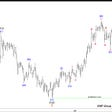## [Leet Code] Move Zeroes## Magic Methods in Python, by example## Dockerizing a Flask-MySQL app with docker-compose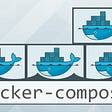## Elrond and HOPR Deepen Partnership## Store and use your SSH password securely for git on Windows## SSAB — September 7 2021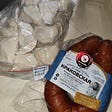## Steven Syrek

High functioning, mostly functional programmer. Words here, codes at github.com/sjsyrek.

## PVS-Studio to help with schoolwork-like tasks in C and C++